Next: Ionization Rate Estimation Up: Analysis of the Particle Previous: The AAGCM Coordinate System

## Conductivity Estimation

The auroral zone conductances can be estimated from the energy flux and average energy of precipitating electrons. The DMSP satellite measures the electron spectral distribution as described in section 4.1 and these measurements can be converted, using several methods, into conductances of the E-layer. A simple expression relating the energy flux and the average energy of the electrons with the height-integrated Hall and Pedersen conductances was described by Robinson et al. [RVM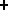87]. The approach makes the assumption that the conductances produced by an actual electron energy distribution can be approximated by those produced by particles with a Maxwellian energy distribution having the same energy flux and average energy.

The average energy of precipitating particles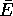is computed according to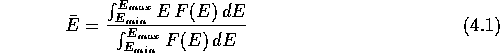where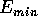and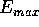are the minimum and maximum energies measured by the detector and F(E) is the differential electron flux. In practice the calculation is performed on the discrete energy channels using the trapezoidal rule for numeric integration. With this average energyand the energy fluxof the electrons the following expressions can be used to determine the Hall conductance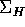and Pedersen conductance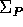: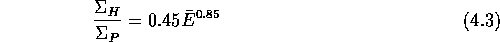whereis measured in keV,in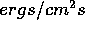,andin mhos. A correction factor of up to 2.0 has to be applied for large average energies when the spectrum is measured below an energy level that is comparable to the average energy. Alsohas to be chosen sufficiently high for the assumption of a Maxwellian distribution to hold;is a good limit for terminating the integration. Comparisons have shown that these estimations yield conductivities that are within 25% of the actual values for most common types of auroral energy distributions [RVM87, p. 2568,]. It is also important to note that averaged auroral conductivity estimates have a much smaller magnitude since they are despiked by the averaging process. Also, for actual conductivity values one has to take the conductivity produced by solar radiation into account. This depends on the level of solar power flux at, denoted by, and the solar elevation angle. The solar radiation conductivity contributions are estimated by Hardy et al. [HGRM87] to be: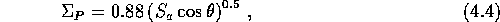Since the spatial variation of the conductivity produced by the solar radiation is small for SuperDARN target area sizes and therefore of little importance for this work, these conductivities are not considered for the data analysis [HGRM87]. There is also evidence that the conductances resulting from photoionization can not be easily modeled using the solar zenith angle and the solar 10.7cm radio flux as scaling parameters. At zenith angles of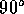the solar radiation components of the Pedersen and Hall conductances are systematically overestimated [WdN92].

The ions have not been taken into account in the conductivity calculations. This is justified in most cases, since in general the ion flux is two orders of magnitude lower than the electron flux. Furthermore, there exist no simple models to include the ion precipitation in conductivity models, while existing complex models which do account for ions have to be refined and checked experimentally [BJSD93].Next: Ionization Rate Estimation Up: Analysis of the Particle Previous: The AAGCM Coordinate System

Andreas Schiffler
Wed Oct 9 10:05:17 CST 1996